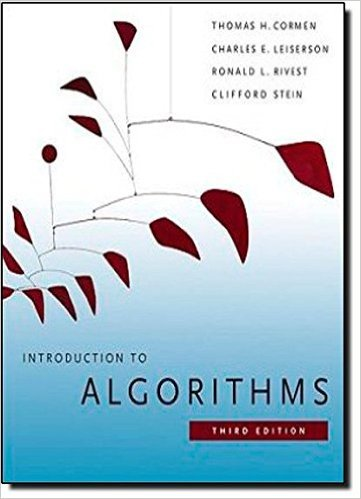×
Get Full Access to Introduction To Algorithms - 3 Edition - Chapter 21 - Problem 21-3
Get Full Access to Introduction To Algorithms - 3 Edition - Chapter 21 - Problem 21-3

×

# Tarjans off-line least-common-ancestors algorithm TheISBN: 9780262033848 130

## Solution for problem 21-3 Chapter 21

Introduction to Algorithms | 3rd Edition

• Textbook Solutions
• 2901 Step-by-step solutions solved by professors and subject experts
• Get 24/7 help from StudySoup virtual teaching assistantsIntroduction to Algorithms | 3rd Edition

4 5 1 353 Reviews
13
5
Problem 21-3

Tarjans off-line least-common-ancestors algorithm The least common ancestor of two nodes u and in a rooted tree T is the node w that is an ancestor of both u and and that has the greatest depth in T . In the off-line least-common-ancestors problem, we are given a rooted tree T and an arbitrary set P D ffu; gg of unordered pairs of nodes in T , and we wish to determine the least common ancestor of each pair in P. To solve the off-line least-common-ancestors problem, the following procedure performs a tree walk of T with the initial call LCA.T:root/. We assume that each node is colored WHITE prior to the walk. LCA.u/ 1 MAKE-SET.u/ 2 FIND-SET.u/:ancestor D u 3 for each child of u in T 4 LCA./ 5 UNION.u; / 6 FIND-SET.u/:ancestor D u 7 u:color D BLACK 8 for each node such that fu; g 2 P 9 if :color == BLACK 10 print The least common ancestor of u and is FIND-SET./:ancestor a. Argue that line 10 executes exactly once for each pair fu; g 2 P. b. Argue that at the time of the call LCA.u/, the number of sets in the disjoint-set data structure equals the depth of u in T . c. Prove that LCA correctly prints the least common ancestor of u and for each pair fu; g 2 P. d. Analyze the running time of LCA, assuming that we use the implementation of the disjoint-set data structure in Section 21.3.

Step-by-Step Solution:
Step 1 of 3

a t\.+ftI -.,-,. ibr^J^\$u,6ske J>6;;tglts/u _- APpttA,ii o{ru; ; Tnc*os,no, an/D

Xr rly+ADZ{G,) _.*_ x, reeAtn :-La*-kob* X1 XL CtrT* y',qL/onG,A-) C+,D *U-,t) D iTf {bco 6 /*.iaC*,Orb b; tsd.o-cNlJnan (a-,6) i )*,sb*;;Ci,:b) r{-@po Wr=* e*,r(a C*,{ca) C^,fe+) lVt^rrb; Att ch^goin[kD occ4;a*cr,h;"4 Mr*,fu-ts,

Step 2 of 3

Step 3 of 3

##### ISBN: 9780262033848

Unlock Textbook Solution